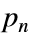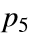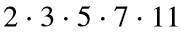# Problem 46054. Count trailing zeros in a primorial

Cody Problem 44068 asked us to count the trailing zeros in a factorial. This problem deals with the primorial. Ifis the nth prime number, then the primorial# is the product of the prime numbers up to and including. For example, if n = 5, then# == 2310, which has one trailing zero.
Count the trailing zeros in the primorial#. Because primorials become large quickly, for large n it helps to derive a formula for the number of trailing zeros.*
*;-)

### Solution Stats

81.08% Correct | 18.92% Incorrect
Last Solution submitted on Nov 03, 2023

### Community Treasure Hunt

Find the treasures in MATLAB Central and discover how the community can help you!

Start Hunting!